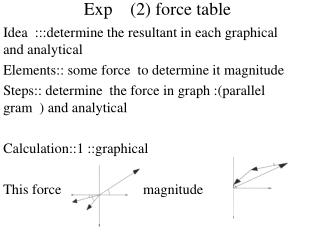DownloadDownload PresentationExp (2) force table

# Exp (2) force table

Télécharger la présentation## Exp (2) force table

- - - - - - - - - - - - - - - - - - - - - - - - - - - E N D - - - - - - - - - - - - - - - - - - - - - - - - - - -
##### Presentation Transcript

1. Exp (2) force table Idea :::determine the resultant in each graphical and analytical Elements:: some force to determine it magnitude Steps:: determine the force in graph :(parallel gram ) and analytical Calculation::1 ::graphical This force magnitude

2. F1 =15n 60 15 30 F2 =8n • In analytical Fx = f1cos60 –f2cos 15 –f3cos30 = 15*0.5-8*0.96-10*0.86=-8.78 n - for direction Fy =f1sin60-f2sin15-f3sin30 =15*0.86-8*0.258-10*0.5=5.9n F mag=Fx+Fy =-8.78+5.9=-2.88n direction is tan-1=Fx =8.78/5.9=1.488== @ =81.86 F3 =10n Fy

3. Results :::Force table We will have three mass on a table like this We will remove the mass until the cycle be in the median and we will take our notes in sketch

4. F1 =1.25*9.8=1.2 F2=2.50*2.45=6.125 F2 =2.50*2.45=6.125